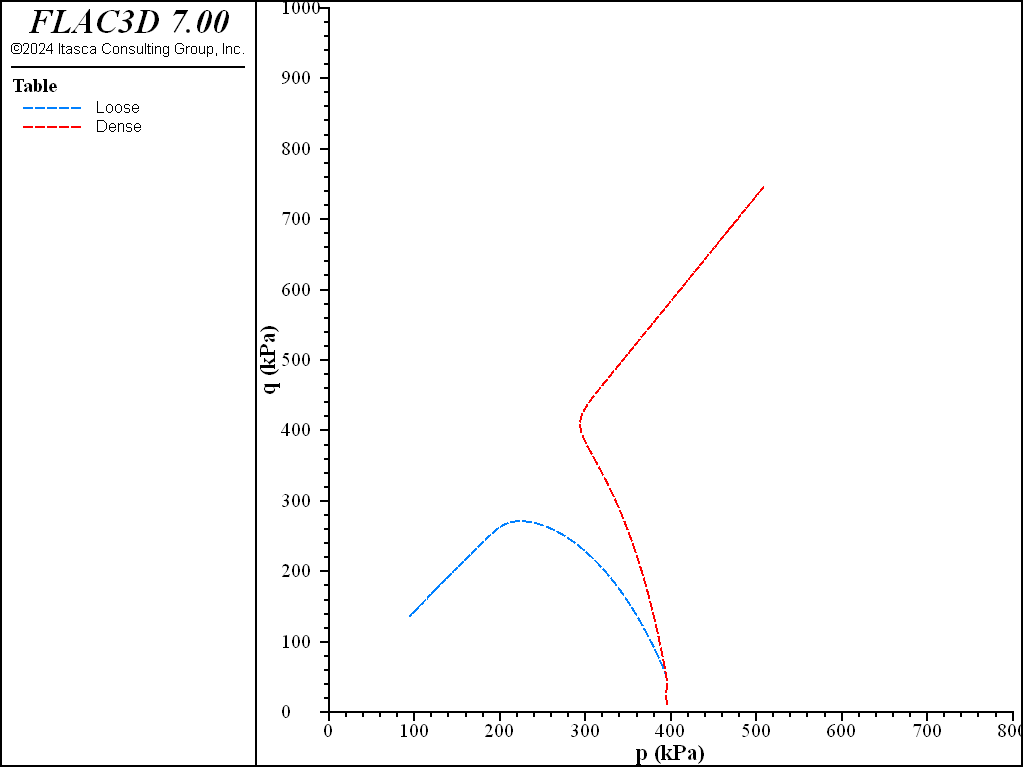FLAC3D Theory and Background • Constitutive Models

# Undrained Triaxial Test with NorSand Model

Note

The project file for this example is available to be viewed/run in FLAC3D. The project’s main data files are shown at the end of this example.

The NorSand model is used to simulate the triaxial consolidated-undrained (CU) tests with with the initial isotropic stress at 395 kPa and OCR = 1.1 without and with the additional softening term ($$S$$ = 0 and 1). The initial state parameters are $$\psi_0=-0.025$$ and $$\psi_0=0.10$$. The material properties are listed in Table 1. Note that in these tests, the state-parameter-dependent hardening modulus ($$H \ne 0$$) and nonlinear stress dependent elastic shear modulus ($$m \ne 1$$) are adopted. Also, a slightly over-consolidated ratio ($$OCR = 1.1$$) is used. The input parameters are summarized in the table:

 $$C_1$$ 1.2 $$C_2$$ 0.06 $$C_3$$ 0.0 $$S$$ 0 and 1 $$M_{tc}$$ 1.47 $$\chi_{tc}$$ 3.2 $$N$$ 0.5 $$H_0$$ 75 $$H_y$$ 400 $$G_{ref}$$ 20970 $$m$$ 0.47 $$p_{ref}$$ 100 $$\nu$$ 0.15 OCR 1.1

Figure 1 and Figure 2 plot the results of simulation by FLAC3D for $$\psi_0=-0.025$$ (dense side of the critical state line) and $$\psi_0=0.10$$ (loose side of the critical state line), respectively. The shear hardening for dense sand and shear softening for loose sand are captured with the same parameters and loading conditions except the initial void ratios.

breakFigure 1: Simulation of $$q$$ vs. axial strain by FLAC3D for undrained triaxial compression tests for initial $$\psi_0=-0.025$$ and $$\psi_0=0.10$$.Figure 2: Simulation of $$q$$ vs. $$p$$ by FLAC3D for undrained triaxial compression tests for initial $$\psi_0=-0.025$$ and $$\psi_0=0.10$$.

Data File

Dense sand datafile:

model new
model large-strain off
model configure fluid
model fluid active off
;
[global psi = -0.025]
;
zone create brick size 1 1 1
zone face skin
zone cmodel assign norsand
;
zone property density 1.6
zone property shear-reference 2.097e4 exponent 0.47 poisson 0.15
zone property c-s-1 1.2 c-s-2 0.06
zone property ratio-critical 1.47 hardening-0 75.0 hardening-y 400.0
zone property factor-dilatancy 3.2 factor-coupling 0.5
zone property state-parameter-initial [psi] o-c-r 1.1
;
zone initialize stress xx -395 yy -395 zz -395
;
program call "ini-stress"
[ini_stress(::zone.list, "norsand")]
;
zone fluid cmodel assign isotropic
zone fluid property porosity 0.3
zone gridpoint initialize fluid-tension -1e20
zone gridpoint initialize fluid-modulus 2e8
;
[global _zvel = -2e-6]
zone face apply velocity-z 0.0     range group 'bottom'
zone face apply velocity-z [_zvel] range group 'top'
zone face apply stress-normal -395  range group 'east' ...
or 'west' or 'north' or 'south'
;
[global _z1 = zone.near(0.5,0.5,0.5)]
fish define q1
local sxx = zone.stress.xx(_z1)
local syy = zone.stress.yy(_z1)
local szz = zone.stress.zz(_z1)
global q1 = sxx - szz
global p1 = -(sxx+syy+szz)/3. - zone.pp(_z1)
global eps_a1 = -gp.disp.z(gp.near(1,1,1))*100. ; axial strain (%)
end
;
fish history name '1' q1
fish history name '2' p1
fish history name '3' eps_a1
;
history interval 50
model cycle 100000
;
history export '1' vs '2' table "dense_qp"
table "dense_qp" export "dense_qp" truncate
history export '1' vs '3' table "dense_qe"
table "dense_qe" export "dense_qe" truncate
;
model save "dense"


Loose sand datafile:

model new
model large-strain off
model configure fluid
model fluid active off
;
[global psi = 0.10]
;
zone create brick size 1 1 1
zone face skin
zone cmodel assign norsand
;
zone property density 1.6
zone property shear-reference 2.097e4 exponent 0.47 poisson 0.15
zone property c-s-1 1.2 c-s-2 0.06
zone property ratio-critical 1.47 hardening-0 75.0 hardening-y 400.0
zone property factor-dilatancy 3.2 factor-coupling 0.5
zone property state-parameter-initial [psi] o-c-r 1.1
;
zone initialize stress xx -395 yy -395 zz -395
;
program call "ini-stress"
[ini_stress(::zone.list, "norsand")]
;
zone fluid cmodel assign isotropic
zone fluid property porosity 0.3
zone gridpoint initialize fluid-tension -1e20
zone gridpoint initialize fluid-modulus 2e8
;
[global _zvel = -2e-6]
zone face apply velocity-z 0.0     range group 'bottom'
zone face apply velocity-z [_zvel] range group 'top'
zone face apply stress-normal -395  range group 'east' ...
or 'west' or 'north' or 'south'
;
[global _z1 = zone.near(0.5,0.5,0.5)]
fish define q1
local sxx = zone.stress.xx(_z1)
local syy = zone.stress.yy(_z1)
local szz = zone.stress.zz(_z1)
global q1 = sxx - szz
global p1 = -(sxx+syy+szz)/3. - zone.pp(_z1)
global eps_a1 = -gp.disp.z(gp.near(1,1,1))*100. ; axial strain (%)
end
;
fish history name '1' q1
fish history name '2' p1
fish history name '3' eps_a1
;
history interval 50
model cycle 100000
;
history export '1' vs '2' table "loose_qp"
table "loose_qp" export "loose_qp" truncate
history export '1' vs '3' table "loose_qe"
table "loose_qe" export "loose_qe" truncate
;
model save "loose"


Endnotes

  To view this project in FLAC3D, use the program menu. Help ▼ Examples…   ⮡   FLAC     ⮡   ConstitutiveModels       ⮡   UndrainedTriaxialNorSand         ⮡   undrainedTriaxialNorSand.prj